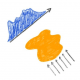###### Comparison of tomography reconstruction techniques
09/08/2018###### Principal Components Regression vs Ridge Regression on NIR data in Python
10/19/2018Not every data point is created equal, and NIR spectroscopy is no different. It is a normal experience, when building a calibration model, to find points that are out of whack with the rest of the group. If  a handful of points doesn’t fit with the rest, it’s fair to call them outliers and remove them from the calibration set. In this post we are going to write Python code to detect outliers with PLS regression applied to NIR spectroscopy.

Well, first we are going to explain the conventional metrics that are used to detect outliers, and then how to implement these metrics in our Python code. This post will build on content I’ve published earlier on PLS regression.

Relevant topics are at these posts.

OK, now we are ready to dive in.

## Metrics to identify outliers with PLS regression

How do we decide if a data point is an outlier? In a very intuitive sense, think of data points in a scatter plot, as in the figure below. It doesn’t take long to intuitively understand that outliers are points which do not follow the general trend, whatever that may be.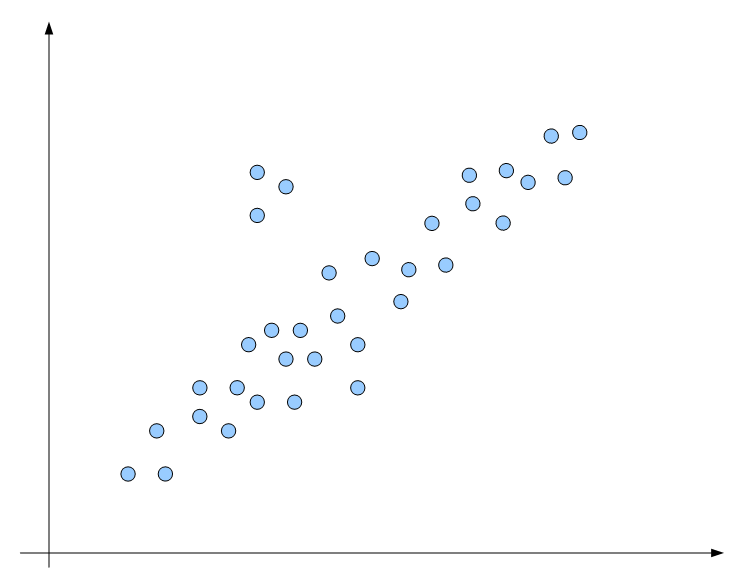In the context of PLS regression (but this is valid for PCA and PCR as well) outliers will be the data points that are not well described by the model.

To make this statement more quantitative I am going to use an example in PLS regression. I’m going to explain a little matrix algebra try and make this as easy as possible. If you really can’t be bothered, feel free to skip to the next section which contains the code. No offence taken.

## A little matrix algebra

In general multivariate PLS, the model for the spectra is written as:

$$X = T \times P^{T} + E$$

To visualise this operation let’s look at the following picture.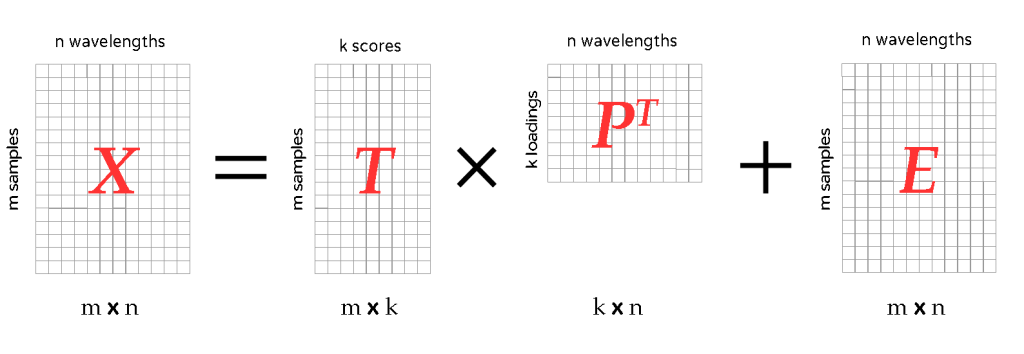• $$X$$ are initial (or preprocessed) spectra. In total we’ve got m spectra (or samples) and n wavelengths.  If we stack them all up we have a  $$m \times n$$ matrix.
• $$P$$ is the loading matrix, which says how much every wavelength band weighs in in the final model. The number k is the number of components we chose for our PLS regression. Finally note that $$P$$ has a superscript: $$P^{T}$$. That just means that we have to transpose the matrix $$P$$ to make the multiplication work.
• The matrix $$T$$ is called the scores. Loosely speaking the scores can be interpreted as a measure of how well each sample is described by the model.
• Finally there is an error matrix $$E$$, which contains all spectral variations in X that cannot be described by the model.

Also, keep in mind that in PLS, a similar model is also built for the response variables, that are the ones we assign as labels, for instance value of Brix associated with a spectrum from a fruit sample. Just for the record, the model for the responses is written as

$$Y = U \times Q^{T} + F$$

where $$Y$$ are the responses and the other matrices are analogous to what we described above. The PLS model is built in such a way to maximise the covariance between $$T$$ and $$U$$.

After this tedious but necessary preamble, we are ready to define the main metrics to detect outliers in PLS. These metrics are generally called with the rather cryptic names of Q-residuals and Hotelling’s T-squared. Let’s try and make them familiar.

## Q-residuals

Q-residuals are derived from the error matrix. Q-residuals account for the variations in the data that are not explained by the model as built.

An outlier is a point that does not follow the general trend of most (or all) other points. That means that for any given model, an outlier will have large Q-residual when compared to the corresponding residuals of the other points.

Q-residuals are calculated in practice by taking the sum of squares of each row of the error matrix. Python code to calculate error matrices and Q-residuals are below.

## Hotelling’s T-squared

Hotelling’s T-squared is the second important metric to detect outliers. While Q-residuals look at the variations that are not explained by the model, T-squared look at the variations within the model itself. A measure of how good is a sample within the model is given by the scores. Low scores mean very good fit. Hotelling’s T-squared can be intuitively thought as a distance of each sample from the ‘ideal’ zero-score situation of perfect fit.

The mathematical definition of this metric is a bit more involved, and I will go easy on you guys here. Suffices to say that the Hotelling’s T-squared is calculated by summing the squares of the rows of the scores matrix T, after normalising each by its standard deviation.

The sum over the rows of the matrix T correspond to summing over the number k of PLS components. Some more details over this procedure is in Kevin Dunn’s excellent book Process Improvement Using Data in the section about Hotelling’s T-squared. I hope the mechanics of this calculations will become clear when we write the code down.

# Outliers detection with PLS in Python

In this section we are going to work through the code required to calculate Q-residuals, Hotelling’s T-squared, and to define a criterion to decide whether a data point is or not an outlier.

We will be using the rice spectral data we already encountered in our previous post on Partial Least Square regression in Python. In that occasion we worked through a calibration-validation approach. Here we will consider a cross-validation approach to evaluate the performance of the calibration model.

The sample data is contained int he array X with dimensions mxn (m=516 is the number of samples, and n=100 is the number of wavelengths). We will used the second derivative, X2, to build the calibration model.

Following a similar procedure as described in the Partial Least Square article, the best model using the full spectrum and the entire set of scans, is obtained by considering 14 latent variables or PLS components). This is our starting point: Q-residuals and Hotelling’s T-squared is first calculated using scores and loading of this basic model

Let’s go explain the main lines step by step. The error array is calculated directly using a little algebra of the PLS formula above: $$E = X – T \times P^{T}$$. In Python the matrix product $$T \times P^{T}$$ is calculated using the numpy  dot  function: np.dot(T,P.T).

To get the Q-residuals, we simply evaluate the sum over the rows of the error array.

For the Hotelling’s T-squared we follow the definition and sum the rows of the score matrix pls.x_scores_, each divided by its standard deviation np.std(pls.x_scores_, axis=0).

The next step to detect outliers is to evaluate the number of points that lie outside a given confidence level, say 95%. This is the most obscure part, and requires a bit of maths and stats to be properly justified. In the spirit of keeping things simple, I won’t be explaining everything in details, but point to the relevant references for those of you who are interested in digging deeper.

This calculation for Hotelling’s T-squared is a bit obscure. The explanation, if you are mathematically inclined, is that the T-squared is distributed according to the F-distribution.  The formula (adapted from the Wikipedia page linked before) is

$$T^{2}_{km} \approx \frac{km}{m-k+1}F_{k,m-k+1}$$

And the percent point function f.ppf(...) evaluates the value of the variable at the given confidence level.

In the absence of a reference distribution, the 95% confidence level for the Q-residuals is estimated by calculating the largest Q-residual, and proceeding backwards. The confidence level Q_conf is the value that is larger than approximately 95% of the Q-residuals.

The last step we may want to do is build a scatter Q-residuals VS Hotelling’s T-squared which marks the position of the confidence level in both axes.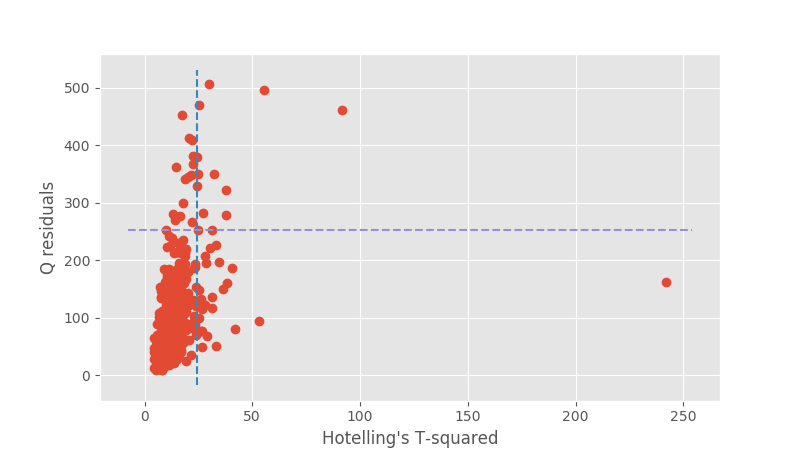A few dozens points out of the 516 we started with fall outside the 95% confidence levels (marked with dashed lines) in either direction. Each point corresponds to the spectrum of one sample. Just to repeat again the main concept, points with large Q-residuals are the ones that are not well explained by the calibration model. Points with large Hotelling’s T-squared values are instead those who display deviations within the model.

# Eliminating outliers to improve the calibration model

The scatter plot above is very useful to get a visual understand of how good our model is to describe the data, and whether there are obvious outliers that could be eliminated to improve the overall predictive power of the calibration model.

Intuitively, with a quick glance at the scatter plot above, we can guess that our model could be likely improved by getting rid of a few points. In this section we are going to write some code to do just that.

We estimate the predictive ability of our model by considering the mean square error in cross-validation. The idea is to remove one outlier at a time up to a maximum value, and  to keep track of the mean square error. We then estimate the optimal number of points to remove by finding the minimum of the mean square error.

Here’s the code

To test the performance of our code, let’s compare the PLS model built with the entire dataset, and the one built by excluding the outliers.

Here’s the model with the entire dataset and its parameters: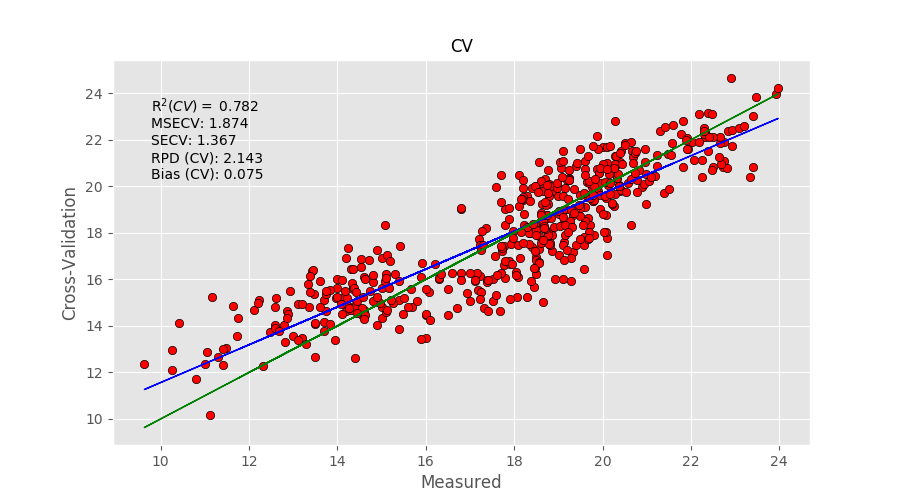And here’s after removing the outliers.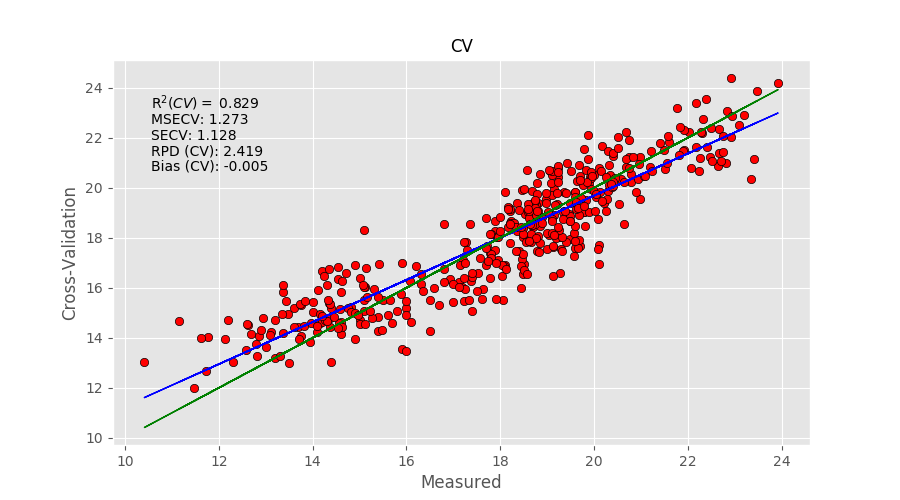The algorithm finds a minimum in the mse cross-validation when 68 outliers are removed. That amounts to a good 30% improvement in the MSE by removing about 13% of the points. Notice how most of the points lie at the lower end of the measured range, that could imply some physical limit to the accuracy in that range.

Thanks for reading and until next time!##### Daniel Pelliccia
Physicist and an entrepreneur. Founder and Managing Director at Instruments & Data Tools, specialising in optical design and analytical instrumentation. Founder at Rubens Technologies, the intelligence system for the fresh fruit export industry.